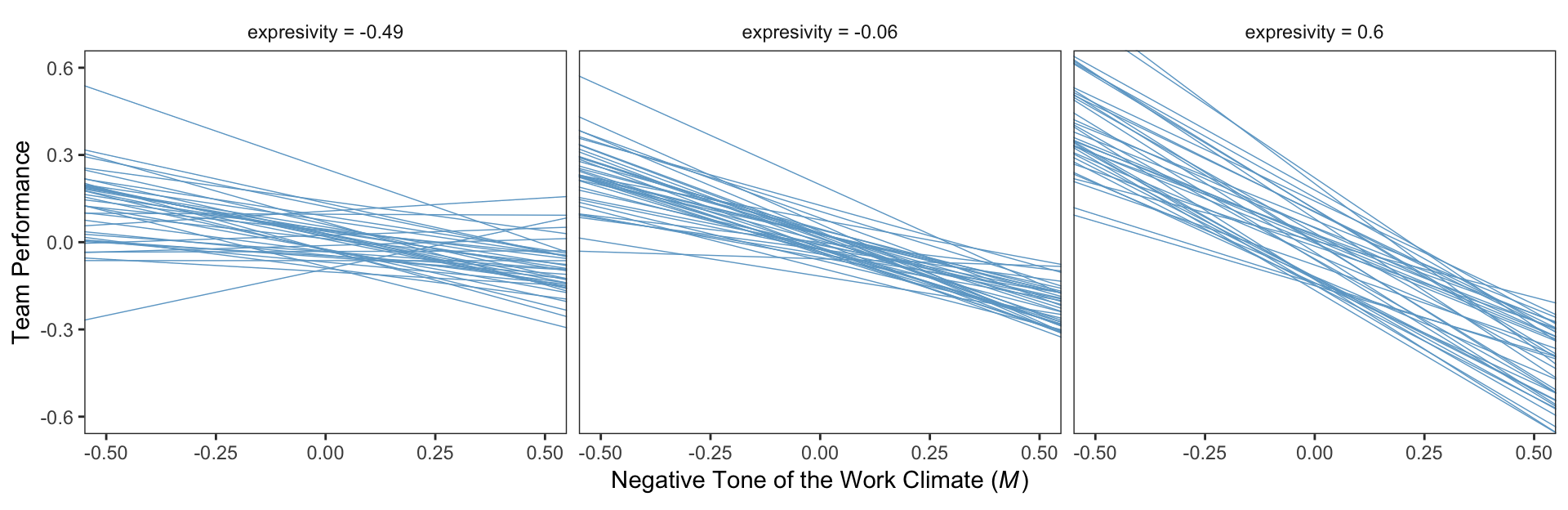## 11.3 Example: Hiding your feelings from your work team

Here we load a couple necessary packages, load the data, and take a glimpse().

library(tidyverse)

teams <- read_csv("data/teams/teams.csv")

glimpse(teams)
## Observations: 60
## Variables: 4
## $dysfunc <dbl> -0.23, -0.13, 0.00, -0.33, 0.39, 1.02, -0.35, -0.23, 0.39, -0.08, -0.23, 0.09, -0.29, -0.... ##$ negtone <dbl> -0.51, 0.22, -0.08, -0.11, -0.48, 0.72, -0.18, -0.13, 0.52, -0.26, 1.08, 0.53, -0.19, 0.1...
## $negexp <dbl> -0.49, -0.49, 0.84, 0.84, 0.17, -0.82, -0.66, -0.16, -0.16, -0.16, -0.16, 0.50, 0.84, 0.5... ##$ perform <dbl> 0.12, 0.52, -0.08, -0.08, 0.12, 1.12, -0.28, 0.32, -1.08, -0.28, -1.08, -0.28, -0.28, -0....

Load the brms package.

library(brms)

Recall that we fit mediation models in brms using multivariate syntax. In previous attempts, we’ve defined and saved the model components outside of the brm() function and then plugged then into brm() using their identifier. Just to shake things up a bit, we’ll just do all the steps right in brm(), this time.

model1 <-
brm(data = teams, family = gaussian,
bf(negtone ~ 1 + dysfunc) +
bf(perform ~ 1 + dysfunc + negtone + negexp + negtone:negexp) +
set_rescor(FALSE),
chains = 4, cores = 4)
print(model1, digits = 3)
##  Family: MV(gaussian, gaussian)
##   Links: mu = identity; sigma = identity
##          mu = identity; sigma = identity
## Formula: negtone ~ 1 + dysfunc
##          perform ~ 1 + dysfunc + negtone + negexp + negtone:negexp
##    Data: teams (Number of observations: 60)
## Samples: 4 chains, each with iter = 2000; warmup = 1000; thin = 1;
##          total post-warmup samples = 4000
##
## Population-Level Effects:
##                        Estimate Est.Error l-95% CI u-95% CI Eff.Sample  Rhat
## negtone_Intercept         0.026     0.062   -0.099    0.147       4000 1.000
## perform_Intercept        -0.011     0.060   -0.131    0.104       4000 1.000
## negtone_dysfunc           0.619     0.166    0.290    0.951       4000 0.999
## perform_dysfunc           0.369     0.184    0.006    0.730       4000 1.000
## perform_negtone          -0.440     0.134   -0.707   -0.178       4000 1.000
## perform_negexp           -0.022     0.120   -0.260    0.213       4000 1.000
## perform_negtone:negexp   -0.512     0.246   -0.992   -0.035       4000 1.000
##
## Family Specific Parameters:
##               Estimate Est.Error l-95% CI u-95% CI Eff.Sample  Rhat
## sigma_negtone    0.487     0.048    0.403    0.592       4000 1.000
## sigma_perform    0.460     0.045    0.383    0.557       4000 0.999
##
## Samples were drawn using sampling(NUTS). For each parameter, Eff.Sample
## is a crude measure of effective sample size, and Rhat is the potential
## scale reduction factor on split chains (at convergence, Rhat = 1).

Our model summary coheres nicely with Table 11.1 and the formulas on page 409. Here are the $$R^2$$ distribution summaries.

bayes_R2(model1) %>% round(digits = 3)
##            Estimate Est.Error  Q2.5 Q97.5
## R2_negtone    0.192     0.077 0.047 0.344
## R2_perform    0.322     0.077 0.166 0.461

On page 410 we get two sample means. Here they are:

mean(teams$negexp) %>% round(digits = 3) ##  -0.008 mean(teams$perform) %>% round(digits = 3)
##  -0.032

For our Figure 11.4 and other similar figures in this chapter, we’ll use spaghetti plots. Recall that with a spaghetti plots for linear models, we only need two values for the variable on the x-axis, rather than the typical 30+.

nd <-
tibble(dysfunc = mean(teams$dysfunc), negtone = rep(c(-.8, .8), times = 3), negexp = rep(quantile(teams$negexp, probs = c(.16, .50, .84)),
each = 2))

Here’s our Figure 11.4, which uses only the first 40 HMC iterations for the spaghetti-plot lines.

fitted(model1,
newdata = nd,
resp = "perform",
summary = F) %>%
as_tibble() %>%
gather() %>%
mutate(iter = rep(1:4000, times = 2*3),
negtone = rep(rep(c(-.8, .8), times = 3),
each = 4000),
negexp = rep(rep(quantile(teams\$negexp, probs = c(.16, .50, .84)),
each = 2),
each = 4000)) %>%
mutate(negexp = str_c("expresivity = ", negexp)) %>%
mutate(negexp = factor(negexp, levels = c("expresivity = -0.49", "expresivity = -0.06", "expresivity = 0.6"))) %>%
filter(iter < 41) %>%

ggplot(aes(x = negtone, y = value, group = iter)) +
geom_line(color = "skyblue3",
size = 1/4) +
coord_cartesian(xlim = c(-.5, .5),
ylim = c(-.6, .6)) +
labs(x = expression(paste("Negative Tone of the Work Climate (", italic(M), ")")),
y = "Team Performance") +
theme_bw() +
theme(panel.grid = element_blank(),
strip.background = element_rect(color = "transparent", fill = "transparent")) +
facet_wrap(~negexp)Also, the plot theme in this chapter is a nod to John Kruschke.